American Journal of Physical Chemistry
Volume 4, Issue 6, December 2015, Pages: 42-47

United Probabilistic Nature and Model of Chemical and Mechanical Reactions of Consecutive Destruction of Substance

Vitaliy Pavlovich Malyshev, Astra Mundukovna Makasheva, Yuliya Sergeevna Zubrina

Chemical and Metallurgical Institute, Karaganda, Republic of Kazakhstan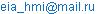(V. P. Malyshev)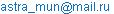(A. M. Makasheva)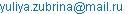(Y. S. Zubrina)

Vitaliy Pavlovich Malyshev, Astra Mundukovna Makasheva, Yuliya Sergeevna Zubrina. United Probabilistic Nature and Model of Chemical and Mechanical Reactions of Consecutive Destruction of Substance.American Journal of Physical Chemistry.Vol.4, No. 6, 2015, pp. 42-47. doi: 10.11648/j.ajpc.20150406.11

Abstract: Into development of mathematical methods of forecasting of grinding process materials based on different aspects of the application of systems theory, the authors propose to be likened to and summarize chemical and mechanical processes of sequential destruction of matter on the basis of a single probability of their nature. Expression for the rate of direct reaction of substances is opened taking into account sense of product of the mole fractions of reacting molecules as probabilities of their simultaneous presence at any point of reactionary space (a concentration factor Pconc), a steric factor of Pst – as probabilities of successful mutual orientation of molecules, an activation factor of Pa – as probabilities of overcoming of a power barrier of activation under the influence of the frequency of impacts of Z: V=ZPconc∙ Pst ∙ Pа. Probabilistic representation of the rate of chemical reactions more directly reflects randomized the state of the reacting system and can be generalized to any of its variants, in particular, mechanical. This allowed us to consider the process of grinding material from new point of view, and moreover - to liken of its kinetics successive irreversible reactions to give the general expression for the output of the intermediates (fractions) at any time for any number of destruction stages. On this basis calculated the entropy of mixing of fractions and the dynamics of change corresponding to the log-normal distribution of fractions which known by data of practices.

Keywords: Chemical Reaction, Mechanical Reaction, Probability Theory, Destruction of the Substance, Ball Mill, Grinding

1. Introduction

There are many papers for various approaches to mathematical description of the processes of crushing, and made the comparative analysis of these approaches [1-7]. They refer to the different aspects of the theory of systems, which adequate complexity of the consideration process, however, requires the identification of a plurality of parameters for obtained the mathematical models on the basis of specific experiments. One of the latest developments in this area is devoted to the prediction of fractional composition of the crushing material on the basis of the principle of maximum entropy  however, this principle can’t be applied to dynamic systems, only to equilibrium.

In this regard, the authors propose a new approach based on the similarity and generalization of the kinetics of chemical and mechanical processes in their understanding of probability and at the same time in strict subordination to of successive transformations of matter to regularities loss and accumulation of each fraction. For this purpose it is necessary to strengthen the probabilistic interpretation of chemical reactions, filling each of its kinetic factors by probabilistic content.

The direct chemical reaction rate

aA+bB®cC+dD                            (1)

in traditional notation is expressed as:

V=k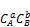,                                (2)

where k – rate constant; СА – concentration of the substance А; СВ – concentration of the substance В; a, b, c, d – stoichiometric coefficients.

We show that in this record essentially veiled probabilistic nature and form of the interaction between matter A and B.

2. Probabilistic Interpretation of the Velocity of Chemical Reaction

As is known, the probability is expressed in fractions of a unit and are dimensionless. Therefore concentration should be represented not in habitual dimensions of g/l, and in molar shares, nA and nB.

Probabilistic meaning mole fraction is that it is essentially the likely presence of a given substance at any point of the volume occupied by the molecules of the reaction mixture. In this case, the rate (2) is expressed as:

V=k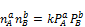(3)

While the mole fractions of nA и nB when raised to a degree, respectively а and в will keep share expression, but the numerical values of nA and nB decrease in nA/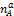=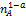and nB/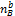=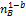time, as always when raising number, the smaller the unit, the power greater than one. But what is the meaning of probabilistic expressions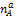and?

It is known that the probability of simultaneous elementary events is expressed as the product of the probabilities of elementary events. Then, to a molecules A and were in the same place in contact with each other, and need a time to multiply the probability of presence in this place molecules of substance A: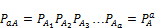(4)

This event in PA/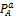=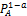times less likely than detection in any point of only one molecule of A.

The same applies to the molecules of the substance of В: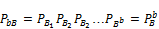(5)

The values obtained cannot be called mole fractions, they are purely probabilistic, which stresses the primary probabilistic meaning of the concept mole fraction.

Following the logic of probability, and the product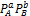should be interpreted as the probability of a joint presence а of molecules A and в of molecules B in the reaction volume anywhere. This value reflects the possibility that the concentration of the reacting molecules in accordance with the stoichiometry of the reaction (1) as a prerequisite for the further development of events, so this value can be defined as a concentration factor, with an possibility of expressing in this way any other reactions: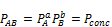(6)

In this case the rate of reaction (3) takes a more universal expression:

V=k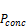(7)

In turn, the constant speed according to the theory of collisions  disclosed as

k=pZ0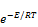(8)

where p – steric (space – orientation) factor; Z0 – collision frequency; E – effective activation energy collisions; R – gas constant; Т – absolute temperature.

It is noted that the steric factor is always less than unity, as it represents the ratio of the mutual orientation of the molecules favorable to the total number of orientations. Thus it makes sense to the probability of mutually successful orientations of molecules and can be considered as a probabilistic steric factor p=Pst.

But the exponentis also mathematically probability between 0 and 1, in the physical sense meaning the probability of overcoming the energy barrier for the conversion of the initial materials into products. This allows to define this as the exponential activation factor

Pа=exp(-E/RT)                               (9)

At last, the frequency of impacts of Z0 sets rate of all probabilistic events which generally has dimension s-1, carried to this or that reactionary volume, and can be designated as Z.

Thus, the equation of the direct reaction rate in the most generalized probabilistic terms can be written as

V=ZPconc∙ Pst∙ Pа                            (10)

It is entirely appropriate all probability factors include both the product (and not, for example, the sum) because they represent successive elementary events are simultaneous contact of reactant molecules, continue their successful mutual orientation, and finally the destruction of the starting material to be converted into products. According to the theory of probability these events are summarized as the product, which is the probability of the entire sequence.

Apart from the most generalized expression of the rate of conversion of the colliding particles it does not contain any restrictions on the mass of these particles and their physical nature. The main thing that a mixture of such bodies was constantly randomized when probability laws manifest themselves most strongly. For molecules such chaotizator is temperature, but rather the thermal kinetic energy of random motion of molecules. But randomization system of colliding bodies can be achieved in any other way, and it can be most clearly illustrated by the grinding of ore in ball drum mills operating in the waterfall (cataract) mode [10-12].

3. Probabilistic Interpretation of the Velocity of Grinding of Materials

With regard to the ball mill for the collision frequency of each grain can be determined from the angular velocity of rotation of the drum (w, s-1) and its diameter (D, m) based on the so-called movement of the Davis cycle (rising to the top point of rotation and on the circumference drop to the lower point) with the help of the proposed formula (g - gravity, m/s2):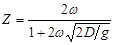, s-1                   (11)

Chance of joint presence at each point of the reaction volume (Pconc) in any case determined by the product of the volume fractions of the reactants, and with respect to the interaction of balls and grains obtained calculation formula [16,17]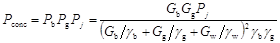(12)

where Рb – the volume fraction of balls in the mixture of ore and water (wet milling); Gb – total mass of balls, kg; gb – density of the material balls, kg/m3; Рg – volume fraction of the ore grains mixed with water and balls; Gg – total mass of ore grains, kg; gg – density of the material grains, kg/m3; Gw – total mass of water in mixture (in pulp), kg; gw – density of water, kg/m3; Pj – the proportion of fractions having an average grain size dj in ore material – and only the target variable in the grinding process. Here the water is a kind of "solvent" or diluents concentration of grinding and grinding bodies.

The corresponding expression has also a steric factor. It depends on a ratio of the geometrical sizes of spheres and grains at sphere blow in a layer of the grains covered with a projection (shadow) of a sphere. Thus in direct contact with a sphere there is only the monolayer clamped between surfaces of a shock platform and a sphere at picture 1.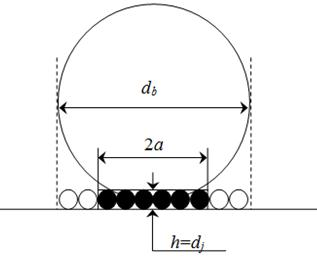db – diameter of the ball, dj –diameter of the grain, h – arrow segment of a sphere

Figure 1. On the calculation of the steric factor. Shaded grain in the fracture zone, not hatched – in a "dead" zone.

This takes only a fraction of a monolayer of the total area under a "shadow" of the ball, taking into account the "dead zone" (not subject to shocks):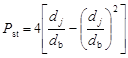(13)

where db and dj – diameters of sphere and grain, m. From this it follows that getting the ball into the grain the less likely the smaller grain size and larger size of the ball, and this explains the first paradox worst grinding small grains of large balls. It is therefore advantageous to add to the large and small balls yet.

But if the ball hit the grain, then the probability of its destruction is the opposite influence of the steric factor, which follows directly from the proposed expression for the activation of factor: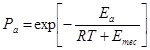(14)

Expressing the impact energy through it turned into potential energy mgh ball drop and attributing it to mole the crushed material (to match the dimensions and RT and Emec), we arrive at a calculation formula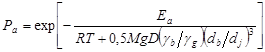(15)

where М – weight average molecular weight substances of ore, kg/mol. Minimum activation energy barrier destruction of grain is its melting heat DHm, J/ mol, which for the main depleting substances, mainly quartz or quartzite, is a reference value.

According to it, the finer the grain and the larger the ball, the greater the probability of failure when hit the ball into the grain. This is the opposite effect of steric factors and activation is illustrated in picture 2 for typical conditions in industrial grinding mills.Figure 2. The dependence of activation and steric factors and their combined effects on the grain size

Based on these data, the highest yield of grain destroyed under the combined action of the Рст and Ра does not exceed a value of about 0, 1 (~ 10%), and this explains the low energy efficiency of the process. At the same time, the fine fractions (10-5-10-6 m) steric factor becomes decisive in the product РstРа, and its proximity to zero (despite the possible one hundred percent destructible from falling into the grain) leads to zeroing РstРа works and thus to the most "grindingless" fines, which remained unexplained.

As a result of the disclosure of all probability factors in equation (1) it is possible to direct the expression rate of decrease in the proportion of the grain Pj size of each dj on these factors, the duration of the process (t, s):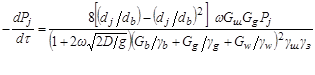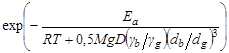, s-1.       (16)

In this formula, time varying quantity is only Pj, so all other parameters can be combined as a constant speed grinding monofraction Pj: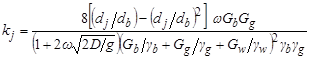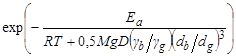, s-1      (17)

and then (16) takes the form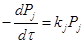, s-1                        (18)

To consider not only a fraction decrease from destruction, but also its replenishment due to receipt of splinters from destruction of the previous fraction, it is required to assimilate, so, and to generalize, process of crushing of grains with kinetics of inreversible consecutive chemical reactions for unlimited number of stages: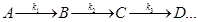(19)

4. Accounting Loss and Accumulation of Fractions in Sequential Destruction of Grain Material

For crushing this sequence of interaction of grains (G) and spheres (B) can be expressed as: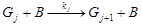(20)

The kinetics of chemical reactions of type (19) is in detail considered and formalized in work . However in it the decision was directed first of all on definition of unknown constants of speeds of chemical reactions. In our case of a constant of speed of crushing pay off on a formula (17), and it is required to find a way out of fractions in each time point. For this purpose system of the differential equations considering an expense and accumulation of each fraction,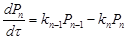(21)

was solved by a method of direct integration with use of integrating multipliers therefore the formula (it is received is probabilistic mathematical model) for an exit n-th fractions taking into account influence on it all previous fractions at any moment [10,14]: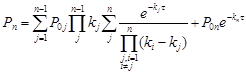(22)

where P0j – initial contents of j fraction.

The calculation of this formula for typical operating conditions of an industrial ball mill gives the following picture of distribution of fractions in logarithmic coordinates (pic. 3).Figure 3. Dependence of fractional structure (Рj, sh.un.) on frequency rate of destruction of grain j and its size dj at increase in duration of process from 60 (1) to 3600 s (2) with according to model (22).

Here also visible during the appearance of double peaks and the area clear logarithmic normal distribution fractions. N. K. Razumovsky paid attention to the last feature in article published still in 1940 in Reports of Academy of Sciences of the USSR on representation of the academician of A. E. Fersman of  and devoted to the analysis of nature of distribution of contents of metals in ore fields for the first time. The conclusions of the author states that the "log-normal, apparently, in the nature of a lot more common than hitherto known. So, it is subject to the distribution of suspended particles in the water, in the air, rock particles from crushing in the chemical deposition ... the presence of this law in the distribution of sediment particles allows to suspect its role in molecular physics. All this makes us look closely at this law ".

Almost immediately responded to this article creator of the theory of probability academician A.N. Kolmogorov . He gave strict probabilistic justification for this law for the case when the rate of fragmentation is independent of particle size, and concludes the paper the following forecast: "It would be interesting to study the mathematical scheme in which the rate of fragmentation of particles decreases (or increases) with the decrease in their size. Naturally in this case to consider primarily the cases in which the speed is proportional to crushing to varying degrees of particle size.

If this degree is other than zero, apparently, logarithmic normal law will be already inapplicable".

But, in a some contradiction with A.N. Kolmogorov's forecast, this law is observed at obvious influence of the size of grains on the constant of the speed recorded by the equation (17), and that the most important – corresponds to numerous practical data. It testifies about objective and more the general character of the developed probabilistic model of crushing and possibility of its use for management of technological process on the basis of expeditious monitoring of all parameters entering a constant of speed, calculation of an exit of target fractions, comparison to their actual distribution and implementation of operating influence by adjustment of controlling parameters.

Equally important discovery proved the relationship between the desire for logarithmic normal distribution fractions and decreasing entropy fractional composition, indicating that the self-organization process of grinding and classification systems to the behavior of its highest complexity [17-20]. With this and the associated long absence of an adequate theory of this process.

5. Сonclusion

In the middle of the XIX century the famous German chemist Lothar Meyer in one of his works suggested that "chemical phenomena should be studied as if they were problems of mechanics" . Judging by the results, the converse is also true: some patterns mechanical interactions can be studied as if they were a branch of chemistry. But correctly to consider their both collectively, recognizing in them action of the system-wide of laws, which subordinate to the theory of probability.

References

1. Kafarov V.V., Dorohov I.N., Arutyunov S.Yu. System analysis of processes of chemical technology. The grinding process of bulk materials. M.: Nauka, 1985. – 440 p.
2. Bernotat S., Schönerl K.: Size Reduction. In: Ullmann’s Encyclopaedia of Industrial Chemistry. VCH, Weinheim, 1998.
3. Andreev S.E., Tovarov V.V., Petrov V.A. Laws of grinding and calculus of characteristics distribution of particle size distribution. – M.: Mettalurgizdat, 1959. – 437 p.
4. Linch A. Cycles of crushing and grinding. – M. Nedra, 1980. – 343 p.
5. Hodakov G.S. Physic of grinding. – M.: Nauka, 1972. – 308 p.
6. The mathematical description and calculation algorithms of mill of cement industry. / Ed. M.A. Verdiyan. M.: NIITSement. – 94 p.
7. L.G. Austin. Introduction to the mathematical description of grinding as a rate process. Powder Technology, 5 (1971/1972) pp. 1-17.
8. Filichev P.V. Forecasting of characteristics of grinding processes through the application of the principle of maximum entropy. Dissertation of the candidate of technical sciences. – Ivanovo, 1999. – 103 p.
9. Emanuel N. M., Knorre D.G. Course of chemical kinetics. The textbook for chemical faculties. Prod. the 3rd, reslave. and additional – M.: The higher school, 1974. – 400 p.
10. Malyshev V.P., Turdukozhayeva A.M. (Makasheva). What Thunder There and is not Heard When Using Ball Mills? // Journal Materials Science and Engineering A. – 2013. – № 2. – V. 3. – P. 131-144.
11. Malyshev V.P., Turdukozhayeva A.M.,Kaykenov D.A. Development of the theory of crushing ores on the basis of the molecular theory of impacts and formal kinetics of consecutive reactions//Ore concentration. – 2012. – №4. – P. 29-35.
12. Malyshev V.P., Turdukozhayeva A.M., Kaykenov D.A. Display of process wet crushing in ball mills probabilistic model//Ore concentration. – 2013. – №1. – P. 27-30.
13. Rodigin N.M., Rodigina E.N. Consecutive chemical reactions. Mathematical analysis and calculation. – M.: Reports of Academy of Sciences of the USSR, 1960. – 140 p.
14. Malyshev V.P., Turdukozhayeva A.M. Kaykenov D. A. Development of mathematical model of consecutive destruction of substance by a method of direct integration// Reports of National Academy of Sciences The Republic of Kazakhstan. – 2012. – №4. – P. 5-13.
15. Razumovsky N.K. The character of distribution of contents metals in ore fields// Reports of Academy of Sciences of the USSR. – 1940. – V. 28. – № 9. – P. 815-817.
16. Kolmogorov A.N. About logarithmic normal law distribution of the sizes at particles when crushing// Reports of Academy of Sciences of the USSR. – 1994. – V.31. – № 2. – P. 99-101.
17. Malyshev V.P., Makasheva A.M., Zubrina Y.S. General view of the integrals in the decomposition of a complex function into elementary fractional. // DNANRK. – 2014. No. 6. – P. 11-14.
18. Malyshev V.P., Zubrina Y.S., Kaikenov D.A., Makasheva A.M. Analysis of convergence and limit of the amount of functional series for fractional composition at the sequential destruction. // DNANRK. – 2015. No. 4. P. 78-83.
19. Malyshev V.P., Turdukozhayeva A.M. Determination of effective energy of activation, the period of semi-crushing and entropy of crushing on the basis of the probabilistic theory of process// Ore concentration. – 2013. – № 5. – P.17-20.
20. Malyshev V.P., Turdukozhayeva A.M., Bekturganov N.S., Kaykenov D A. Logarifmic normal distribution of fractions when crushing materials as an attractor in probabilistic model of process// Reports of National Academy of Sciences The Republic of Kazakhstan. – 2013. – № 6. – P. 46-52.
21. Dickerson R., Gray G., Haight D. Basic laws of chemistry: in 2 volumes. Trans. from English. – M.: Mir. 1982. – 620 p. – V. 2. – P. 350.

 Contents 1. 2. 3. 4. 5.
Article ToolsAbstractPDF(488K)